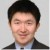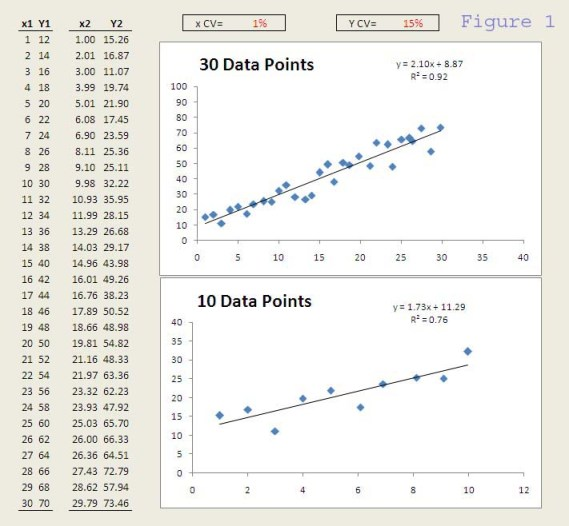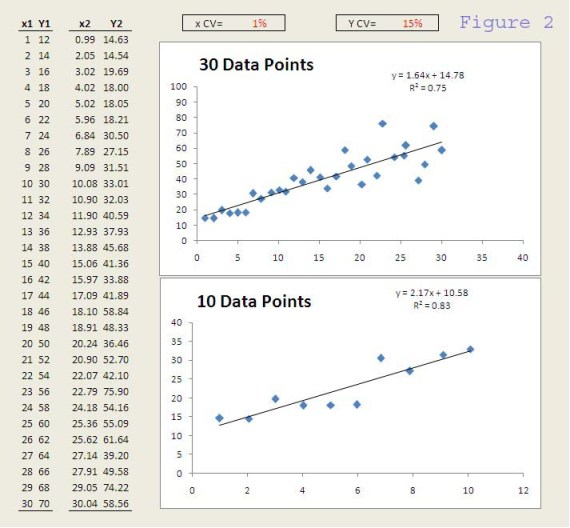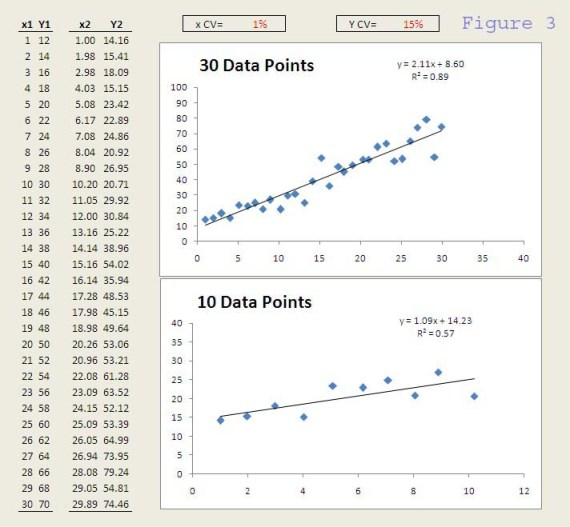# Correlation and Variation

ByFang Zhou

Six Sigma projects differ from traditional projects in one important requirement understanding of the Y=f(x) relationship with data before developing or implementing a solution. In the DMAIC framework, we identify, test and verify the causal relationship between a potential root cause (x) and the outcome (Big Y), and then develop a solution to change the x to move the Y in the desired direction.

This sounds simple enough. But in practice, why is it so hard to identify and/or verify such relationships? Is the concept of hypothesis testing too difficult? Are statistical tools too complicated for the average person? Maybe. But before we teach people statistical tools, we have to first help them develop statistical thinking the concept of variation.

Handpicked Content:   Sensei Sue???

All the statistical tools would be unnecessary if there were no variation in life.

So how does variation affect our ability to understand Y=f(x)? To give an example, I created a simple Excel sheet to show how variation (or noise) can affect our conclusions on whether two variables correlate in a linear regression.The Y=f(x) relationship in this example is Y = 2x + 10.

Without other sources of variation, change in x from 1 to 30 would lead to change in Y from 12 to 70 (as shown by columns x1 and Y1 in Figure 1).

However, if we add 1% of variation to x (or 1% Coefficient of Variation, which is defined as the standard deviation divided by the mean) and 15% variation to Y, the data would be as in columns x2 and Y2.

Now look at the charts made from x2 and Y2 in Figure 1. The top one shows all 30 data points, whereas the bottom one shows only the first 10 data points. The linear regression lines and equations are also shown. Would you conclude that Y and x correlate if you had the data on the top graph? How about the bottom?

What about the data from figure 2 or 3?Did you draw the same conclusion? And you SHOULD because the data sets came from the same underlying system. The differences (in R-square, slope and intercept) we see among the data is due to pure random variation. In this example, I used the random number generator (RAND function) in Excel and a normal distribution (NORMINV function) to generate the random values deviating from the expected x or Y.

Handpicked Content:   How Do You Eat an Elephant?

In a real system, the variation includes all sources (in addition to the x of interest) that can affect the Y. So a 15% CV is fairly small compared to the variation in many real systems. With a 15% CV, about 95% of the time, the data will appear within +/- 30% of the expected value. Even with such small variation, data from a sample (such as those in any of the six graphs) could lead to a different conclusion if statistical thinking is not applied. In problems where variation or uncertainty exists (i.e. practically everything we do), the conclusion should always be expressed/understood in statistical or probabilistic terms.

No matter how sophisticated the statistical tools we use, one goal is common to separate sources of variation so we can understand how the variable of interest affects the outcome. In practice, the challenge often lies in identifying and then reducing undesired sources of variation, i.e. noise. For most people, however, the first step is simply to appreciate how greatly variation impacts our everyday decisions, and to apply statistical thinking.

[Simulations by simple tools, such as Excel, can help us appreciate the impact of variation on our practical decisions. For example, how quickly does increasing variation (larger CV’s) affect the R-square, slope and intercept of the regression? Or how useful is an R-square cutoff (say, >0.7) in deciding if there is a strong correlation?]

##### Fang Zhou

Pharmaceutical/Biotechnology

1.